December 27, 2021

# Class 10 Science Chapter 1 Chemical Reactions And Equations

In this chapter, we will learn about chemical reactions and equations. But, before we go into that, let’s first take a look at some history about the chemical reaction. In the middle ages, chemical reactions were studied by alchemists, they tried to turn lead into gold by using lead and lead-copper (which are alloys with sulfur), they tried all these short of techniques to play with chemistry.

In Class 10 Science Chapter 1 Chemical Reactions and Equations, we will try to do the same This chapter includes all the fun and easy doing experiments, you can easily do all these experiments by yourself, but always stay alert and do these experiments under the supervision of a teacher or someone who can guide you.

## Class 10 Science Chapter 1 Chemical Reactions and Equations

This chapter is all about fun experiments with the chemicals and learning about their reactions and equations. Here we discuss all the important topics in the easiest way possible so that you don’t have to face any difficulty understanding them.

Before going into Class 10 Science Chapter 1 Chemical Reactions and Equations let’s see what have we learned in Class IX about atoms and chemical reactions.

• In the process of chemical reaction sum of the masses of both reactants and products remains unchanged also known as laws of conservation of mass.
• We also learned about the laws of conservation of definite proportions.
• We also learned about the atom that it usually can’t exist independently.
• A molecule is the smallest particle of an element that shows all properties of the substance.
• We learned about the polyatomic ions and they carry fixed charges on them.
• We also learned about the Avogadro constant i.e. 6.022 x 10²³.
• Also, we learned about the molar mass and the mole amount of the substance i.e. 6.02214076 x 10²³.

What are reactions?

Reactions: It occurs when 2 or more molecules interact with each other and they change. Now bonds between them are broken and after that new molecule was formed.

What are Atoms and what are molecules?

Atoms: An atom is the smallest unit of matter that forms a chemical element. Everything is made up of atoms. Everything around you is also made up of atoms like Solid, Liquid, and Gas.

Molecules: Molecules are made up of 2 or more atoms that contain chemical bonds between them.

### Class 10 Science Chapter 1 Chemical Reactions and Equations

In this section, we will learn things in an understandable way to make it easy for you to remember things related to this chapter.

# Chemical Equations

A chemical equation is the symbolic representation of a chemical reaction in the form of symbols and formulae. In other words, we show the chemical reaction between 2 substances by using symbols, you can check the example below.

for example: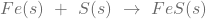Here:

• Fe → Iron
• S → Sulfur
• FeS → Iron Sulfide
• (s) → Solid

In this reaction when we combine Iron with Sulfur it forms another element i.e. Iron Sulfide. However, all the elements which are on the left side of the arrow are known as reactants. And the right side of the arrow is known as the products.

#Chemical Reactions

The chemical reaction is a process in which 1 or more substances are converted to 1 or more substances. You can easily see many reactions in the Class 10 Science Chapter 1 Chemical Reactions and Equations for example:

• Rusting of an Iron: It happens when oxygen reacts with the iron, and they make “rust”.
• Photosynthesis: It happens inside the plants and trees.

There are hundreds of examples of chemical reactions. So, chemical reactions are everywhere and can be found in your day-to-day life.

# Balancing the chemical equation

Sometimes, the chemical equations are not balanced which means the quantity of the substances on both sides of the arrow is not equal. So, sometimes you have to balance the equation by adding numbers on either side. Let’s understand this through an example.

Example:

There are several steps you have to follow in order to balance the equation. See below:

Equation: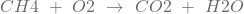Element LHS Quantity RHS Quantity
Oxygen (O) 2 3
Hydrogen (H) 4 2

STEP 1: Check which elements need to be balanced, like in this case Oxygen (O) and Hydrogen (H) needs to be balanced.STEP 2: Now, multiply 2 in H2O to make Hydrogen (H) balanced. So, now both sides have 4 Hydrogen (H). And now we have to balance the quantity of Oxygen too,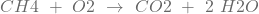STEP 3: In this step, we multiply O2 by 2 so that the quantity of oxygen becomes equals on both sides.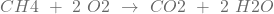So, this chemical equation now become balanced. As you can see the quantity of all the chemical elements is now equal on both sides.

Element LHS Quantity RHS Quantity
Oxygen (O) 4 4
Hydrogen (H) 4 4

#### Class 10 Science Chapter 1 Chemical Reactions and Equations

Here we provide all the detailed information about the types of chemical reactions and equations.

# Types of Chemical Reactions

There are 5 types of chemical equations. These are Combination reaction, Decomposition reaction, Displacement reaction, Double Displacement reaction, and Oxidation & Reduction. So, down in the article, we explain all these types in detail.

1. Combination Reaction

It is a reaction where 2 or more elements combine to form a single element. Let’s understand it with the help of an example below:
Equation: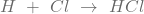Here:

• H → Hydrogen
• Cl → Chlorine
• HCl → Hydrogen Chloride

In this equation, 2 elements i.e. Hydrogen (H) and Chlorine (Cl) combine together to make new element Hydrogen Chloride (HCl).

While you experiment with all these reactions you may find that the reaction mixture becomes warm. This is because in some of the reactions along with the product element heat is also released. And these types of chemical reactions are known as Exothermic reactions. See the example below:

Equation :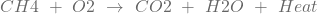2. Decomposition Reaction

In this chemical reaction, 1 element or reactant breaks into 2 or more elements or products are known as a decomposition reaction. Let’s take a very simple example:

Equation: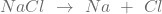Here:

• NaCl → Sodium Chloride
• Na → Sodium
• Cl → Chlorine

In this equation, we can see that Sodium Chloride commonly known as Salt is a combination of Sodium and Chlorine. Now, you must be thinking that how can we break a single element into 2 different elements.

So, for breaking an element we need some energy in a form of heat, light, or electricity. Although, those reactions in which some kind of energy is absorbed are also known as endothermic reactions.

#### Class 10 Science Chapter 1 Chemical Reactions and Equations

Now let’s see more types of chemical reactions that are included in chapter 1 of class 10th.

3. Displacement Reaction

In this reaction, the atom or group of atoms that are present in the molecule or element is replaced by another atom is known as displacement reaction. Let’s understand this with an example.

Equation: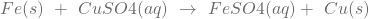Here:

• Fe → Iron
• Cu → Copper
• FeSO → Iron Sulfate
• CuSO → Copper Sulfate
• (s) → Solid
• (aq) → Liquid

In this reaction, when we add Iron (Fe) to the copper sulfate (CuSO) solution it displaces the Copper (Cu) from it and makes a new element i.e. Iron sulfate (FeSO).

4. Double Displacement Reaction

In this reaction, the 2 elements or reactants react by exchange of ions between them and give us 2 new elements or products. Let’s understand it with an example.

Equation: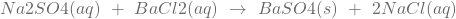Here:

• Na → Sodium
• SO → Sulfur Oxide
• Ba → Barium
• Cl → Chloride
• NaSO → Sodium Sulphate
• BaCl → Barium Chloride
• BaSO → Barium Sulphate
• NaCl → Sodium Chloride

In this reaction, there is an insoluble substance formed which is also known as a precipitate. As in the equation given above, we can see that when we combine Sodium sulfate with Barium chloride it gives the product element i.e. Barium sulfate, and a precipitate which is Sodium Chloride also known as Salt.

5. Oxidation and Reduction

Oxidation and Reduction are known as adding and removing oxygen elements from a compound.

Oxidation: It means addition of oxygen in the equation. See the example below:

Equation: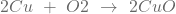In this reaction after the combination of 2 reactants, there is an addition of oxygen Cu, which makes CuO.

Reduction: It means the subtraction of oxygen from the equation.

Equation: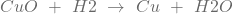In this reaction after the combination of 2 reactants, there is a subtraction of oxygen from the CuO element and it is added in Hydrogen to make H2O.

#### Class 10 Science Chapter 1 Chemical Reactions and Equations

In this section, we are going to learn about the effects of oxidation reactions in everyday life.

# Effects of Oxidation Reactions in everyday life

There are mainly 2 types of effects caused by the oxidation reaction. You can see these types of effects in your everyday life.

# Corrosion

You can see this reaction mostly on the Iron surface or on things made up of Iron. Although, this happens to sulfur and copper too. You must have noticed that something made of Iron is very shiny in the beginning, but if you leave that thing in an open environment for a while, then the color of Iron starts to turn brown. Which means that thing catches rust.

This is known as corrosion on the Iron. The same thing can happen with Sulfur as it turns black in color and with Copper, it turns green in color. We will discuss this in more detail in Chapter-3.

# Rancidity

Have you ever noticed that the food which is kept in open without any cover gets spoiled as time passes? It also happens with fruits, raw vegetables, and cooked food.

It happens because the oxidation combines with fats and oils of food. After this, the taste and smell of the food become very bad. Because of this, we have to cover our food and store it in the refrigerator so that it doesn’t spoil. This oxidation reaction which combines with fats and oils is known as rancidity.

Class 10 Science Chapter 1 Chemical Reactions and Equations
3 Marker 1 question
Total questions 1
Total marks 3
Class 10 Science Chapter 1 Chemical Reactions and Equations Blueprint 2021-22

The total worth of Class 10 Science Chapter 1 Chemical Reactions and Equations in terms of the board exam is 3 marks for 1 question. Please note that this marking scheme may vary in board exams.

Hopefully, you liked our article on Class 10 Science Chapter 1 Chemical Reactions and Equations. If you have any doubt feel free to write us in the comment section.